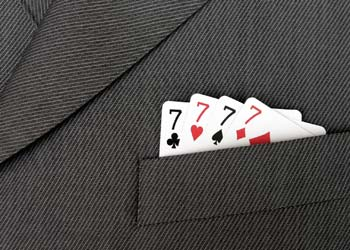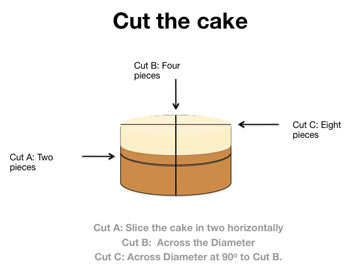# Interesting and Amazing Math Facts

By Nick Valentine| Last update: 21 October 2019

The more one studies mathematics, the more mysterious it becomes, with powers that seem quite 'spooky' and almost magical at times.Consider the Power of Pi: it seems such a simple concept, the ratio between the circumference of a circle and its diameter. As a fraction, that's simply 22 over 7 but as an actual number, Pi is unknowable.

See the box for an approximate (!) statement of the value of Pi but in fact you could go on calculating it into eternity and never find a pattern or reach the end. So we just call it 3.142.

But consider how this "irrational" number seems to crop up everywhere. Pi is all over the natural world, wherever there's a circle, of course, measuring patterns in the DNA double helix spiral or how ripples travel outward in water. It helps describe wave patterns or the meandering patterns of rivers.

π = 3.14159 26535 89793 23846 26433 83279 50288 41971 69399 37510 58209 74944 59230 78164 06286 20899 86280 34825 34211 70679 82148 08651 32823...

But Pi isn't just connected with circles. For example, the probability that any two whole numbers among a random collection are "relatively prime" with no common factor is equal to 6 over Pi squared. Pi even enters into Heisenberg's Uncertainty Principle; the equation that defines how precisely we can know the state of the universe.

So Pi is just one example of the 'magic' of math. If you want more proof of this, consider the following:

## Pi and pizzas are linked

You multiply Pi multiplied by the radius squared to find the area and multiply area by height to find the volume, That means the volume of a pizza that has a nominal radius of (z) and height (a) will, of course, be: Pi × z × z × a

And strangely, if you enter Pi to two decimal places (3.14) in the your calculator and look at it in the mirror, you'll see it spells 'pie'.

## Nature loves Fibonacci sequences

The spiral shapes of sunflowers and other patterns in nature follow a Fibonacci sequence, where adding the two preceding numbers in the sequence gives you the next (1, 1, 2, 3, 5, 8, etc.)

## In a crowded room, two people probably share a birthday

It only takes 23 people to enter a room to give you an evens chance that two of them have the same birthday. With 75 people in the room the chances rise to 99 per cent!

## Multiplying ones always gives you palindromic numbers

If you multiply 111,111,111 × 111,111,111 you get 12,345,678,987,654,321 - a palindrome number that reads the same forwards or backwards. And that works all the way back down to 11 x 11 (121) or just 1 x 1 (1).

## The universe isn't big enough for Googolplex

A googolplex is 10 to the power of a googol, or 10 to the power of 10 to the power of 100. Our known universe doesn't have enough space to actually write that out on paper. If you try to do that sum on a computer, you'll never get the answer, because it won't have enough memory.

## Seven is the favorite numberYou might have guessed that most people's favorite number is 7 but that's now been proven.

A recent online poll of 3,000 people by Alex Bellos found that around 10% of them chose seven, with three as the runner-up.

That might be because seven has so many favorable connections (seven wonders of the world, pillars of wisdom, seven seas, seven dwarves, seven days, seven colors in the rainbow). But it's also true that seven is "arithmetically unique" - the only single number you can't multiply or divide while keeping the answer within the 1-10 group.

## Prime numbers help Cicadas survive

Cicadas incubate underground for long periods of time before coming out to mate. Sometimes they spend 13 years underground, sometimes 17. Why? Both those intervals are prime numbers and biologists now believe cicadas adopted those life-cycles to minimize their contact with predators with more round numbered life-cycles.

On the next page we look at how the answer is always 6174, how random patterns aren't really random and we reveal 14 other snap maths facts.

## The answer is always 6174

Starting with any four digit number (that has at least two different digits) just follow the following steps:

1. Arrange the digits of the four digit number in descending/ascending order to make the largest and smallest numbers possible.
2. Subtract the smaller number from the larger one.
3. Take the answer and repeat the process.

Eventually you'll end up at 6174 or 'Kaprekar's Constant'. Just as remarkable, it never takes more than seven stages to get there.

Picking a number at random, let's try 4551, for instance.

Stage 1: 5541-1455 = 4086
Stage 2: 8640 - 0468 = 8172
Stage 3: 8721 - 1278 = 7443
Stage 4: 7443 - 3447 = 3996
Stage 5: 9963 - 3699 = 6264
Stage 6: 6642 - 2466 = 4176
Stage 7: 7641 - 1467 = 6174

## 1, 2, 3, 4, 5, 6, 7, 8, 9 make 100

... but not with those comma placements. There are at least three different ways to use the numbers 1-9 in that order without multiplying or dividing in order to reach 100:

Route 1:
123 + 4 - 5 + 67 - 89 = 100.

Route 2:
123 - 4 - 5 - 6 - 7 + 8 - 9 = 100.

Route 3:
1 + 23 - 4 + 5 + 6 + 78 - 9 = 100.

Bet you can find Route 4...

## Random patterns aren't really random

Weirdly, random numbers aren't actually all that random. In a given list of numbers representing anything from populations to building heights to border lengths, fully one third of them will begin with the digit 1. Fewer will begin with 2 and so on until only one number in twenty begins with a 9. The bigger the data set, and the more orders of magnitude it spans, the more strongly this pattern emerges.

## 0.999... = 1

How can 1 equal 0.999? Well, it does, and we can prove that in two different ways.

Proof 1:

If N = 0.999, then 10N = 9.99.

10N - N is therefore 9.99 - 0.999 therefore 9N = 9 therefore N =1

Proof 2:

If N = 0.999 then N divided by 9 is 0.111

Express this as the equation:

• 0.111 = 1/9

Multiplying both sides by 9 produces:

• 0.999 = 1

What's going on here? In two words, 'decimal expansion'. 0.999 really represents 0.999999999 and on ad infinitum with each place to the right of the decimal point representing a further negative power of 10.

So the decimal expansion 0.9999... actually represents the sum 9/10 + 9/100 + 9/1000. Adding a further place of decimals (0.9999) would add just 9/10000 and so on into infinity until the two values are so close as to be indivisible.

## Snap maths facts1. You can cut a cake into eight equal pieces with just three straight cuts. Give up? Take a look at the box at the end of the article for the illustration of how to do it.
2. Adding up the numbers 1-100 consecutively (1+2+3+4+5...) gives you 5050.
3. Shuffle a pack of cards really thoroughly and theres'a greater chance than not that the exact sequence in the deck has never been seen before in all of recorded history.
4. 2 and 5 are the only primes that end in 2 or 5.
5. From 0 to 1,000, the letter "A" only appears in 1,000 ("one thousand").
6. A 'jiffy' is an actual unit of time. It means 1/100th of a second.
7. 'FOUR' is the only number in the English language that is spelt with the same number of letters as the number itself.
8. 40 when written "forty" is the only number with letters in alphabetical order, while "one" is the only one with letters in reverse order.
9. The numeral 4 is associated in Japanese and Chinese cultures with 'death' (Many Chinese hospitals do not have a 4th floor).
10. A circle has the largest area of any shape with the same perimeter.
11. A circle also has the shorted perimeter of any shape with the same area.
12. The Greek father of maths, Pythagoreans, used little rocks to represent equations. numbers. Hence calculus, which is the Ancient Greek word meaning 'pebbles'. The word "fraction" derives from the Latin fractio "to break".
13. At sixes and nines, the result of the sum (6 × 9) + (6 + 9) is... 69. How about that?
14. Getting back to Pi, one way to remember its shortened value (3.1415926) is to count the letters in each word of the question: 'May I have a large container of coffee?'

Do you know any more fascinating maths facts? If so, please feel free to leave them in the comments box below and we can discuss them.